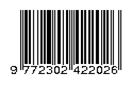### Rectilinear Monotone r-Regular Planar Graphs for r = {3, 4, 5}

Arthur Wulur, Benny Pinontoan, Mans Mananohas

#### Abstract

A graph G consists of non-empty set of vertex/vertices (also called node/nodes) and the set of lines connecting two vertices called edge/edges. The vertex set of a graph G is denoted by V(G) and the edge set is denoted by E(G). A Rectilinear Monotone r-Regular Planar Graph is a simple connected graph that consists of vertices with same degree and horizontal or diagonal straight edges without vertical edges and edges crossing. This research shows that there are infinite family of rectilinear monotone r-regular planar graphs for r = 3and r = 4. For r = 5, there are two drawings of rectilinear monotone r-regular planar graphs with 12 vertices and 16 vertices.

Keywords: Monotone Drawings, Planar Graphs, Rectilinear Graphs, Regular Graphs

#### Full Text:

PDF

DOI: https://doi.org/10.35799/dc.4.1.2015.8318

### Refbacks

• There are currently no refbacks.

Indexed By: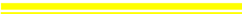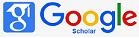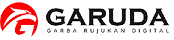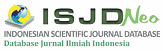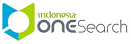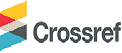e-ISSN: 2685-1083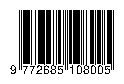p-ISSN: 2302-4224